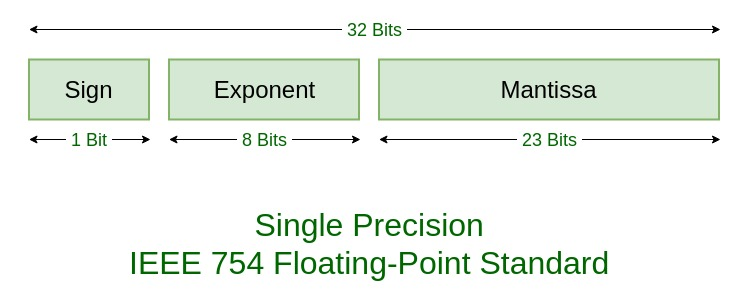# Program for conversion of 32 Bits Single Precision IEEE 754 Floating Point Representation

Pre-Requisite: IEEE Standard 754 Floating Point Numbers

Write a program to find out the 32 Bits Single Precision IEEE 754 Floating-Point representation of a given real value and vice versa.Examples:

```Input: real number = 16.75
Output: 0 | 10000011 | 00001100000000000000000

Input: floating point number = 0 | 10000011 | 00001100000000000000000
Output: 16.75
```

Approach:
This implementation is based on Union Datatype in C and using the concept of Bit Fields.

Bit Fields are assigned when we don’t require the full memory that is usually allocated to some variables but we want to limit the amount of memory taken up by those variables. In C, members of a Union share the common memory space and taken we can access the members only one at a time.

Below is the implementation of the above approach:

Program 1: Convert a real value to its floating point representation

 `// C program to convert a real value ` `// to IEEE 754 floating point representaion ` ` `  `#include ` ` `  `void` `printBinary(``int` `n, ``int` `i) ` `{ ` ` `  `    ``// Prints the binary representation ` `    ``// of a number n up to i-bits. ` `    ``int` `k; ` `    ``for` `(k = i - 1; k >= 0; k--) { ` ` `  `        ``if` `((n >> k) & 1) ` `            ``printf``(``"1"``); ` `        ``else` `            ``printf``(``"0"``); ` `    ``} ` `} ` ` `  `typedef` `union` `{ ` ` `  `    ``float` `f; ` `    ``struct` `    ``{ ` ` `  `        ``// Order is important. ` `        ``// Here the members of the union data structure ` `        ``// use the same memory (32 bits). ` `        ``// The ordering is taken ` `        ``// from the LSB to the MSB. ` `        ``unsigned ``int` `mantissa : 23; ` `        ``unsigned ``int` `exponent : 8; ` `        ``unsigned ``int` `sign : 1; ` ` `  `    ``} raw; ` `} myfloat; ` ` `  `// Function to convert real value ` `// to IEEE foating point representation ` `void` `printIEEE(myfloat var) ` `{ ` ` `  `    ``// Prints the IEEE 754 representation ` `    ``// of a float value (32 bits) ` ` `  `    ``printf``(``"%d | "``, var.raw.sign); ` `    ``printBinary(var.raw.exponent, 8); ` `    ``printf``(``" | "``); ` `    ``printBinary(var.raw.mantissa, 23); ` `    ``printf``(``"\n"``); ` `} ` ` `  `// Driver Code ` `int` `main() ` `{ ` ` `  `    ``// Instantiate the union ` `    ``myfloat var; ` ` `  `    ``// Get the real value ` `    ``var.f = -2.25; ` ` `  `    ``// Get the IEEE floating point representation ` `    ``printf``(``"IEEE 754 representation of %f is : \n"``, ` `           ``var.f); ` `    ``printIEEE(var); ` ` `  `    ``return` `0; ` `} `

Output:

```IEEE 754 representation of -2.250000 is :
1 | 10000000 | 00100000000000000000000
```

Program 2: Convert a floating point representation to its real value

 `// C program to convert ` `// IEEE 754 floating point representaion ` `// into real value ` ` `  `#include ` `#include ` ` `  `typedef` `union` `{ ` ` `  `    ``float` `f; ` `    ``struct` `    ``{ ` ` `  `        ``// Order is important. ` `        ``// Here the members of the union data structure ` `        ``// use the same memory (32 bits). ` `        ``// The ordering is taken ` `        ``// from the LSB to the MSB. ` ` `  `        ``unsigned ``int` `mantissa : 23; ` `        ``unsigned ``int` `exponent : 8; ` `        ``unsigned ``int` `sign : 1; ` ` `  `    ``} raw; ` `} myfloat; ` ` `  `// Function to convert a binary array ` `// to the corresponding integer ` `unsigned ``int` `convertToInt(``int``* arr, ``int` `low, ``int` `high) ` `{ ` `    ``unsigned f = 0, i; ` `    ``for` `(i = high; i >= low; i--) { ` `        ``f = f + arr[i] * ``pow``(2, high - i); ` `    ``} ` `    ``return` `f; ` `} ` ` `  `// Driver Code ` `int` `main() ` `{ ` ` `  `    ``// Get the 32-bit floating point number ` `    ``unsigned ``int` `ieee ` `        ``= { 1, ` `            ``1, 0, 0, 0, 0, 0, 0, 0, 0, 0, 1, ` `            ``0, 0, 0, 0, 0, 0, 0, 0, 0, 0, 0, ` `            ``0, 0, 0, 0, 0, 0, 0, 0, 0 }; ` ` `  `    ``myfloat var; ` ` `  `    ``// Convert the least significant ` `    ``// mantissa part (23 bits) ` `    ``// to corresponding decimal integer ` `    ``unsigned f = convertToInt(ieee, 9, 31); ` ` `  `    ``// Assign integer representation of mantissa ` `    ``var.raw.mantissa = f; ` ` `  `    ``// Convert the exponent part (8 bits) ` `    ``// to a corresponding decimal integer ` `    ``f = convertToInt(ieee, 1, 8); ` ` `  `    ``// Assign integer representation ` `    ``// of the exponent ` `    ``var.raw.exponent = f; ` ` `  `    ``// Assign sign bit ` `    ``var.raw.sign = ieee; ` ` `  `    ``printf``(``"The float value of the given"` `           ``" IEEE-754 representation is : \n"``); ` `    ``printf``(``"%f"``, var.f); ` `} `

Output:

```The float value of the given IEEE-754 representation is :
-2.250000
```

My Personal Notes arrow_drop_upIf you like GeeksforGeeks and would like to contribute, you can also write an article using contribute.geeksforgeeks.org or mail your article to contribute@geeksforgeeks.org. See your article appearing on the GeeksforGeeks main page and help other Geeks.

Please Improve this article if you find anything incorrect by clicking on the "Improve Article" button below.